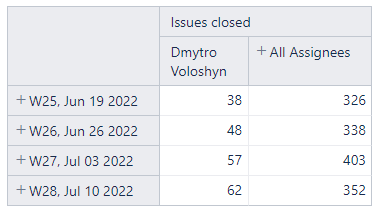# Get average of closed issues per week

I building a report of closed issues per week for all assignees in the project and for one specific team member.
I want to ass new measure - Avg issues closed per week for the assigness that were actually assigned to the issues during the week.

for this table:assuming in the first week there were 10 assignees working this week the number will by 326/10
assuming in the second week there were 15 assignees working this week the number will by 338/15

and so on…
Any idea how???

You can define a new calculated measure “Assignee counter” in the Measures dimension that will iterate through the Assignee list and count the number of Assignees that had an issue assigned to them during the corresponding week in rows.

The formula would be along these lines:

``````Count(
Filter(
DescendantsSet([Assignee].CurrentMember,[Assignee].[User]),
(
[Measures].[Transitions to assignee],
[Assignee].CurrentMember
) > 0
)
)
``````

You can then define a new calculated measure that would divide the two measures:

``````[Measures].[Issues closed]
/
[Measures].[Assignee counter]
``````

Let me know if this fits your use case!

Best regards,
Nauris / eazyBI support